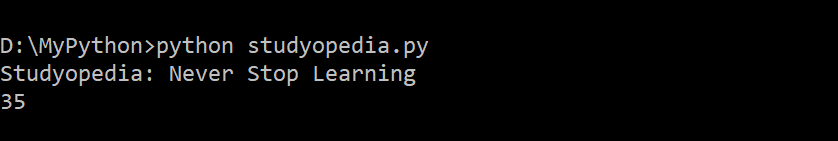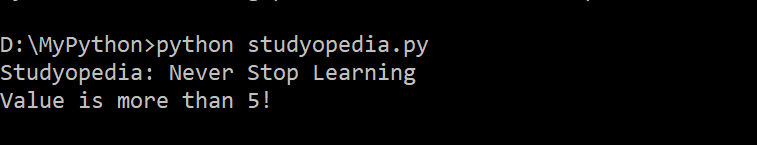# Python Decision Making Statements

## 19 May Python Decision Making Statements

With decision making statement like if, if…else, elseIf, switch, etc, you can easily take decision based on different conditions. In this lesson we will learn how to work with Python Decision Making Statements. In the previous lesson we learned how to work with loops in Python.

## if statement in Python

The if decision making statement in Python executes the code, if the condition is true.

### Syntax

Here’s the syntax,

### Example

The following is an example showing the usage of if statement in Python,

### Output

The following is the output,## if…else in Python

The if…else decision making statement in Python executes some code if the condition is true and another code if the condition is false. The false condition comes under else.

### Syntax

Here’s the syntax,

### Example

The following is an example showing the usage of if…else statement in Python,

### Output

The following is the output,## if…elif…else in Python

The if…elif…else statement in Python execute code for different conditions. If more than 2 conditions are true, you can use else for the false condition.

### Syntax

Here’s the syntax,

### Example

The following is an example showing the usage of if…elif…else statement in Python,

Here, myVar = 8 and the else condition will get executed.

### Output

The following is the output,In this lesson we learned how to work with decision making statements in Python.[email protected]

We work to create free tutorials for all.